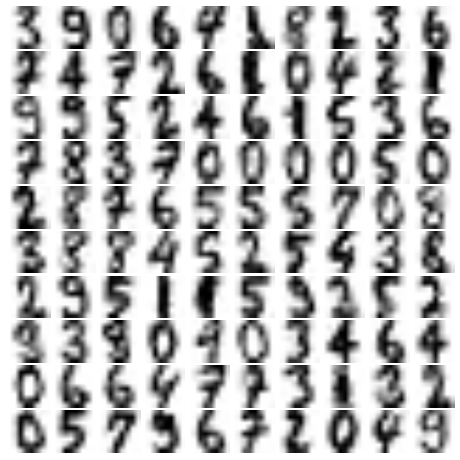Scikit-Learn学习笔记——高斯混合模型(GMM)应用：分类、密度估计、生成模型

高斯混合模型

k-means聚类模型非常简单并且易于理解，但是他的简单性也为实际应用带来了挑战。特别是实际应用中，k-means的非概率性和它仅根据到簇中心点的距离来指派将导致性能低下。高斯混合模型可以看作是k-means的一个扩展，但它也是一种非常强大的聚类评估工具。

高斯混合模型

%matplotlib inline
import matplotlib.pyplot as plt
import seaborn as sns; sns.set()
import numpy as np

#产生实验数据
from sklearn.datasets.samples_generator import make_blobs
X, y_true = make_blobs(n_samples=400, centers=4,
cluster_std=0.60, random_state=0)
X = X[:, ::-1] #交换列是为了方便画图

from sklearn.mixture import GMM
gmm = GMM(n_components=4).fit(X)
labels = gmm.predict(X)
plt.scatter(X[:, 0], X[:, 1], c=labels, s=40, cmap='viridis');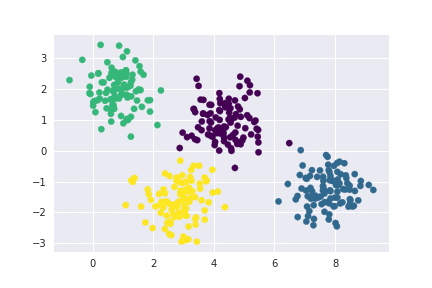#由于GMM有一个隐含的概率模型，因此它也可能找到簇分配的概率结果——在Scikit-Learn中用predict_proba方法
#实现。这个方法返回一个大小为[n_samples, n_clusters]的矩阵，矩阵会给出任意属于某个簇的概率
probs = gmm.predict_proba(X)
print(probs[:5].round(3))

#输出结果
[[0.525 0.475 0.    0.   ]
[0.    0.    0.    1.   ]
[0.    0.    0.    1.   ]
[1.    0.    0.    0.   ]
[0.    0.    0.    1.   ]]
#将每个点簇分配的概率可视化
size = 50 * probs.max(1) ** 2  #平方放大概率的差异
plt.scatter(X[:, 0], X[:, 1], c=labels, cmap='viridis', s=size);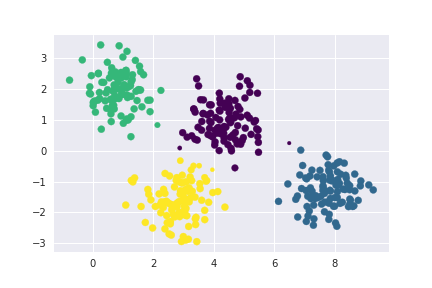使用GMM拟合拟合扁平椭圆的簇

from matplotlib.patches import Ellipse

def draw_ellipse(position, covariance, ax=None, **kwargs):
"""用给定的位置和协方差画一个椭圆"""
ax = ax or plt.gca()

#将协方差转换为主轴
if covariance.shape == (2, 2):
U, s, Vt = np.linalg.svd(covariance)
angle = np.degrees(np.arctan2(U[1, 0], U[0, 0]))
width, height = 2 * np.sqrt(s)
else:
angle = 0
width, height = 2 * np.sqrt(covariance)

#画出椭圆
for nsig in range(1, 4):
ax.add_patch(Ellipse(position, nsig * width, nsig * height,
angle, **kwargs))

def plot_gmm(gmm, X, label=True, ax=None):
ax = ax or plt.gca()
labels = gmm.fit(X).predict(X)
if label:
ax.scatter(X[:, 0], X[:, 1], c=labels, s=40, cmap='viridis', zorder=2)
else:
ax.scatter(X[:, 0], X[:, 1], s=40, zorder=2)
ax.axis('equal')

w_factor = 0.2 / gmm.weights_.max()
for pos, covar, w in zip(gmm.means_, gmm.covars_, gmm.weights_):
draw_ellipse(pos, covar, alpha=w * w_factor)
#用椭圆形来拟合数据
rng = np.random.RandomState(13)
X_stretched = np.dot(X, rng.randn(2, 2))
gmm = GMM(n_components=4, covariance_type='full', random_state=42)
plot_gmm(gmm, X_stretched)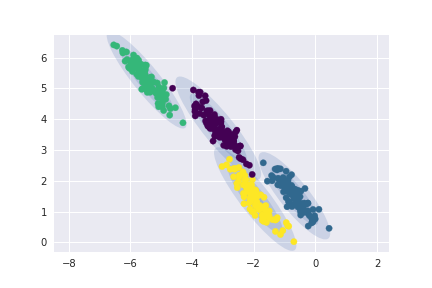GMM模型中的超参数convariance_type控制这每个簇的形状自由度。

• 它的默认设置是convariance_type=’diag’,意思是簇在每个维度的尺寸都可以单独设置，但椭圆边界的主轴要与坐标轴平行。
• covariance_type=’spherical’时模型通过约束簇的形状，让所有维度相等。这样得到的聚类结果和k-means聚类的特征是相似的，虽然两者并不完全相同。
• covariance_type=’full’时，该模型允许每个簇在任意方向上用椭圆建模。

将GMM用作密度估计

#生成实验数据
from sklearn.datasets import make_moons
Xmoon, ymoon = make_moons(200, noise=.05, random_state=0)
plt.scatter(Xmoon[:, 0], Xmoon[:, 1]);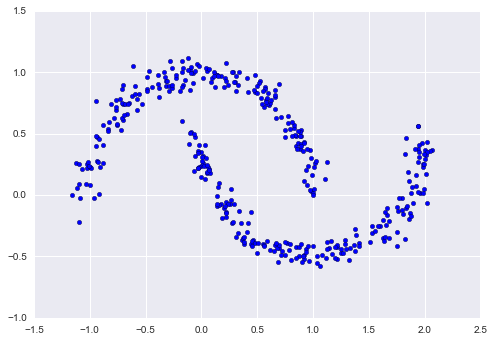#如果用GMM对数据拟合出两个成分，那么作为一个聚类模型的结果，效果将会很差
gmm2 = GMM(n_components=2, covariance_type='full', random_state=0)
plot_gmm(gmm2, Xmoon)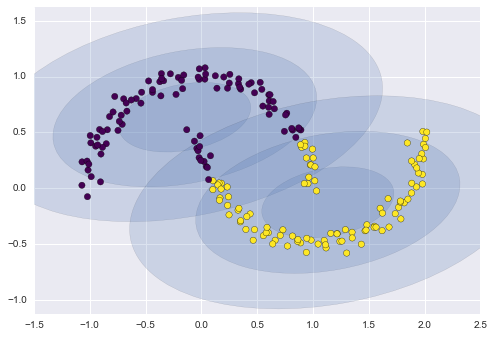#如果选用更多的成分而忽视标签，就可以找到一个更接近输入数据的拟合结果
gmm16 = GMM(n_components=16, covariance_type='full', random_state=0)
plot_gmm(gmm16, Xmoon, label=False)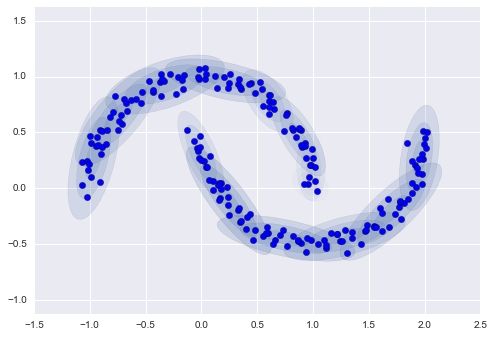Xnew = gmm16.sample(400, random_state=42)
plt.scatter(Xnew[:, 0], Xnew[:, 1]);n_components = np.arange(1, 21)
models = [GMM(n, covariance_type='full', random_state=0).fit(Xmoon)
for n in n_components]

plt.plot(n_components, [m.bic(Xmoon) for m in models], label='BIC')
plt.plot(n_components, [m.aic(Xmoon) for m in models], label='AIC')
plt.legend(loc='best')
plt.xlabel('n_components');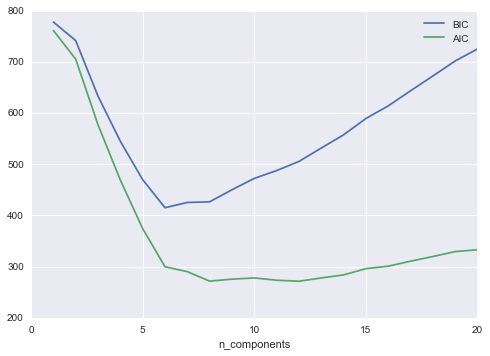示例：用GMM生成新的数据

#导入手写数字数据
digits.data.shape

#画出前100个数据
def plot_digits(data):
fig, ax = plt.subplots(10, 10, figsize=(8, 8),
subplot_kw=dict(xticks=[], yticks=[]))
for i, axi in enumerate(ax.flat):
im = axi.imshow(data[i].reshape(8, 8), cmap='binary')
im.set_clim(0, 16)
plot_digits(digits.data)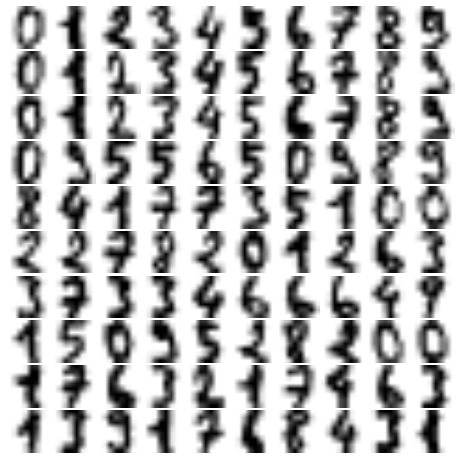#使用PCA进行降维，让PCA算法保留投影后样本99%的方差
from sklearn.decomposition import PCA
pca = PCA(0.99, whiten=True)
data = pca.fit_transform(digits.data)

#对这个降维的数据使用AIC，从而得到GMM成分数量的粗略估计
n_components = np.arange(50, 210, 10)
models = [GMM(n, covariance_type='full', random_state=0)
for n in n_components]
aics = [model.fit(data).aic(data) for model in models]
plt.plot(n_components, aics);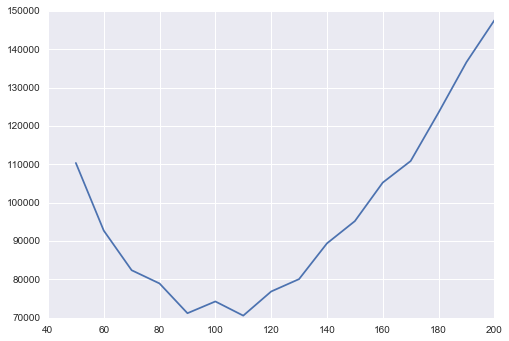#在大约110个成分的时候，AIC是最小的，因此我们打算使用这个模型生成新的数据
#确认模型已经收敛
gmm = GMM(110, covariance_type='full', random_state=0)
gmm.fit(data)
print(gmm.converged_)

#输出结果：
True
#使用GMM模型在降维的空间中画出100个新的手写数字样本，再使用PCA对象逆变换将其恢复到原始的空间
data_new = gmm.sample(100, random_state=0)
digits_new = pca.inverse_transform(data_new)
plot_digits(digits_new)